# Fantastic cats and how to control them

Author - Shinibali Bhattacharyya, Quantum Physicist at Qruise GmbH.

## 1. Introduction

Cat-qubits are a special kind of superconducting qubits where quantum information is encoded into superpositions of multiple Fock states of a harmonic oscillator. Although such ingenious engineering can provide protection against bit-flip errors , creation and manipulation of these qubits require complex quantum gates that need to address multiple Fock states simultaneously.

In this post, we demonstrate how one can model the cat-qubits within the Qruise ecosystem and simulate their dynamics pertaining to state-preparation and measurement. With optimal control of drive pulses, we prepare a cat-qubit that reaches $96\%$ of a targeted photon occupation number of $2.25$, within $4 \,\mu s$ of pumping.

Before we dive in to the Qruise toolset, we would like to walk the reader through a brief hardware schematics of the Alice&Bob cat-qubit set-up from 2020  as shown in the picture below.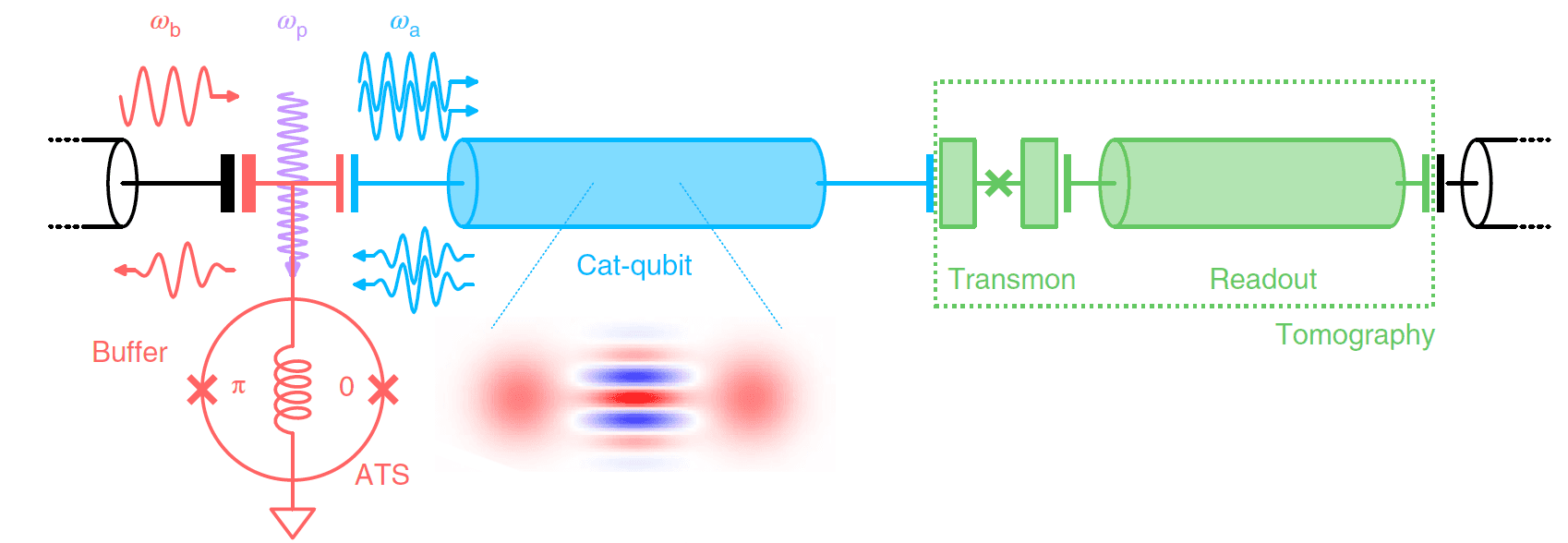Fig.1 Picture adapted from Ref.. The buffer (red) is a lumped element resonator connected to ground through a nonlinear element called asymmetrically threaded SQUID (ATS). The buffer is coupled to the cat-qubit resonator (blue). Pumping the ATS at frequency $\omega_p = 2\omega_a − \omega_b$ (purple arrow), where $\omega_{a,b}$ are the cat-qubit and buffer frequencies, mediates the exchange of two photons of the cat-qubit (blue arrows) with one photon of the buffer (red arrows) and populates the cat-cavity. On the other end, the cat-cavity is coupled to a transmon qubit and a readout resonator (green) to facilitate Wigner tomography for measurements.

## 2. Cat qubits in Qruise toolset

Due to the large Hilbert space of the collective cat-buffer-transmon mode, we will divide the numerics and simulate the problem in two halves: first, open-system dynamics for cat-qubit state preparation using the buffer and cat-cavity, and second, dissipation-less Wigner tomography  for reading out cat-states from coupled cat-transmon modes.

## 2.1. State preparation

The following section simulates the dynamics of a cat-state creation in a cat cavity coupled to a buffer, under the effects of dissipation in both the cavities. The corresponding Hamiltonian in the frame of the cat-cavity and the buffer-mode is:

$\mathbf{H}_{RWA} /\hbar = \omega_{a,0} \mathbf{a^† a} + \omega_{b,0} \mathbf{b^† b} + g_2^* \mathbf{a}^2 \mathbf{b}^† + g_2 \mathbf{a}^{†2} \mathbf{b} - \sum_{m=a,b} \frac{\chi_{mm}}{2}\mathbf{m}^{†2}\mathbf{m}^{2} - \chi_{ab}\mathbf{a}^{†}\mathbf{a}\mathbf{b}^{†}\mathbf{b} + \epsilon_B(t) (\mathbf{b^†} + \mathbf{b}) + \epsilon_C(t) \mathbf{a^†} \mathbf{a}$

where $\mathbf{a/b}$ are annihilation operators of cat/buffer modes, $\omega_{a/b,0}$ their resonant frequencies. $g_2$ is the coupling term connecting the buffer and the cat-cavity, $\chi_{aa/bb}$ is the self-Kerr term (anharmonicity) for the cat/buffer resonator, and $\chi_{ab}$ is the cross-Kerr coupling between them. $\epsilon_B(t)$ is the drive function coupled to the buffer-mode. $\epsilon_C(t)$ is the drive function coupled to the cat-mode to enable Kerr-correction. The coupling $g_2$ enables pair-wise exchange of photons from/to the cat-cavity and the buffer. At resonance, the buffer is driven such that $\omega_{b,0}=0$ and correspondingly, the frequency of the cat-cavity is $\omega_{a,0} =0$. We simulate the effective resonance Hamiltonian:

$\mathbf{H}_{i} /\hbar = g_2^* \mathbf{a}^2 \mathbf{b}^† + g_2 \mathbf{a}^{†2} \mathbf{b} - \sum_{m=a,b} \frac{\chi_{mm}}{2}\mathbf{m}^{†2}\mathbf{m}^{2} - \chi_{ab}\mathbf{a}^{†}\mathbf{a}\mathbf{b}^{†}\mathbf{b} + \epsilon_d(t) (\mathbf{b^†} + \mathbf{b}) + \epsilon_C(t) \mathbf{a^†} \mathbf{a}$

Dissipation is essential to this dynamics, and the loss operators are given by: $\mathbf{L}_a = \sqrt{\kappa_a} \mathbf{a}, \mathbf{L}_b = \sqrt{\kappa_b} \mathbf{b}$ where $\kappa_b >> \kappa_a$. The idea is that the driven buffer (alongwith a pump not explicitly shown in the equations) populates and stabilizes the cat-modes in the cat-cavity against dissipation.

### 2.1.1. Instantiating cat and buffer modes

This setup is an example notebook, providing all necessary details for the curious readers. But one can also get their "digital-twin" set up without copying every tiny details. Having mentioned that, first, we import the relevant modules.

Click to view the module imports
# System imports
import copy
import numpy as np
import tensorflow as tf
import os
import tempfile

# Main Qruise toolset objects
from qruise.toolset.objects import Quantity as Qty
from qruise.toolset.parametermap import ParameterMap as PMap
from qruise.toolset.experiment import Experiment as Exp
from qruise.toolset.model import Model as Mdl
from qruise.toolset.generator.generator import SimpleGenerator
from qruise.toolset.generator.generator import Generator as Gnr

# Building blocks
import qruise.toolset.signal.gates as gates
import qruise.toolset.libraries.components as components
import qruise.toolset.signal.pulse as pulse
import qruise.toolset.generator.devices as devices

# Libs and helpers
import qruise.toolset.libraries.envelopes as envelopes
import qruise.toolset.utils.tf_utils as tf_utils
import qruise.toolset.libraries.algorithms as algorithms

# Libs and helpers from Catqubit library
from qruise.toolset.libraries.catqubit.components import CatStates, Buffer
from qruise.toolset.libraries.catqubit.hamiltonians import hamiltonians
from qruise.toolset.libraries.catqubit.utils import (
coherent_state, plot_evolution, _partial_trace, get_purity_of_state,
get_partial_trace, cat_state_vec, _purity, get_fidelity,
post_select_state, ideal_3level_Ry_gates, _return_dm
)
from qruise.toolset.libraries.catqubit.fidelity import state_overlap_infid, trace_distance_infid

# Qiskit related modules
from qruise.toolset.vis.oscillator import OscillatorStateViewer

# For optimal control
from qruise.toolset.optimizers.optimalcontrol import OptimalControl
from qruise.toolset.optimizers.loggers import PlotLineChart
from qruise.toolset.libraries.algorithms import lbfgs, cmaes


We list the relevant hardware parameters from Alice&Bob cat-qubit chip (2020) :

# Cat cavity parameters
alpha = 1.5 # displacement value |α|^2 = photon number of coherent state
dim_cat = 13 # this is the Fock dimension of cat cavity
freq_cat = 0 # 0 Hz for resonance mode, 8.03805e9 2 pi Hz for alicebob hardware
chi_cat = -7e3 # 2 pi Hz Kerr coupling for cats
kappa_cat = 53e3 # 2π Hz, in other words t1 = 2π/κ_b = 3e-6 s
qubit_temp = 50e-3 # K

# Buffer parameters
dim_buffer = 3 # this is the hilbert dimension of buffer mode
freq_buffer = 0 # 0 Hz for resonance mode, 4.8336e9 2 pi Hz for alicebob hardware
chi_buffer = -32e6 # 2 pi Hz, Kerr coupling for buffer
kappa_buffer = 13e6 # 2π Hz, in other words t1 = 2π/κ_b = 4.833e-7 s

# Transmon parameters
dim_trans = 3 # this is the hilbert dimension of transmon mode
freq_trans = 0 # 0 Hz for stead state cat-buffer frame, 4.4156e9 2 pi Hz for alicebob hardware
chi_trans = 180e6 # 2 pi Hz, Kerr coupling for transmon
t1_trans = 5e-6 # s
t2_trans = 8e-6 # s

# coupling parameters
chi_buff_cat = 0.79e6 # 2 pi Hz, cross-Kerr between buffer and cat
g2_buff_cat = 0.36e6 # 2 pi Hz, photon transfer coupling between buffer and cat
chi_trans_cat = 0.72e6 # 2 pi Hz, cross-Kerr between transmon and cat

# Drive amplitude and time for buffer
driveB_amp = tf.math.real(alpha * tf.math.conj(alpha) * g2_buff_cat )
t_driveB = 4e-6 # 2 mu s square pulse

# Drive amplitude for Kerr-correction on cat cavity:
driveC_kerr_correct = tf.math.real((2.0 * alpha * tf.math.conj(alpha) + 1.0) * chi_cat )

# Resolution for numerical simulation
sim_res = 200e6 # Hz
v2Hz = 1 # used in converting Hz to Volts for generator

# Delay times for transmon mode
t_delay = 0.5 / chi_trans_cat # 6.94e-7 s wait time between two gates = π/χ_qa (χ as Hz) = 0.5/χ_qa (χ as 2π Hz)

# params for envelope for gaussian gate pulses on transmon
awg_res = sim_res/50 # limited resolution of an AWG
sideband = 50e3 # 2 pi Hz
sigma = t_delay/4 # 4 sigma = 8 mu s


Next, we add the cat-cavity Hamiltonian terms $(\omega_{a,0} \mathbf{a^† a} - \frac{\chi_{aa}}{2}\mathbf{a}^{†2}\mathbf{a}^{2})$ using the component class $\textcolor{orange}{\textrm{CatStates}}$:

# Cat mode
cat = CatStates(
name="cat",
desc="Cat mode",
freqa=Qty(value=freq_cat, unit="Hz 2pi"),
chiaa=Qty(value=chi_cat, unit="Hz 2pi"),
hilbert_dim=dim_cat,
kappa=Qty(value=kappa_cat, unit="Hz 2pi"),
temp=Qty(value=qubit_temp, unit="K"),
)


Then, we add the buffer-Hamiltonian terms $( \omega_{b,0} \mathbf{b^† b} - \frac{\chi_{bb}}{2}\mathbf{b}^{†2}\mathbf{b}^{2} )$ using the component class $\textcolor{orange}{\textrm{Buffer}}$:

# Buffer mode
buffer = Buffer(
name="buffer",
desc="Buffer mode",
freqb=Qty(value=freq_buffer, unit="Hz 2pi"),
chibb=Qty(value=chi_buffer, unit="Hz 2pi"),
hilbert_dim=dim_buffer,
kappa=Qty(value=kappa_buffer, unit="Hz 2pi"),
temp=Qty(value=qubit_temp, unit="K"),
)


Next, we set up the coupling term $(g_2^* \mathbf{a}^2 \mathbf{b}^† + g_2 \mathbf{a}^{†2} \mathbf{b})$ that results in creating the cat-states in the cat-cavity.

# Photon exchange coupling between buffer-cat
coupling_cat_buffer = components.Coupling(
name="cat-buffer coupling",
desc="coupling cat and buffer",
connected=["buffer", "cat"],
strength=Qty(value=g2_buff_cat, unit="Hz 2pi"),
hamiltonian_func=hamiltonians["coupling_cat_buffer"],
)


We add the cross-Kerr coupling between the cat and buffer-mode $(\chi_{ab}\mathbf{a}^{†}\mathbf{a}\mathbf{b}^{†}\mathbf{b})$.

# Cross-kerr coupling between buffer and cat cavity
kerr_cat_buffer = components.Coupling(
name="cat-buffer kerr",
desc="cross-kerr cat and buffer",
connected=["buffer", "cat"],
strength=Qty(value=chi_buff_cat, unit="Hz 2pi"),
hamiltonian_func=hamiltonians["cross_kerr"],
)


Next, we set up the drive function $\epsilon_d(t)$ with its associated Hamiltonian term $(\mathbf{b^†} + \mathbf{b})$.

# Driving field for the buffer
drive_buffer = components.Drive(
name="dB",
desc="Drive buffer",
comment="Drive line 1 on buffer",
connected=["buffer"],
hamiltonian_func=hamiltonians["buffer_xdrive_ham"],
)


Next, we set up a drive function $\epsilon_C(t)$ with its associated Hamiltonian term $(\mathbf{a^†} \mathbf{a})$ acting on the cat-cavity as a corrective term for Kerr-effect.

# Driving the cat for Kerr-correction
drive_cat = components.Drive(
name="dC",
desc="Drive cat",
comment="Drive line 2 on cat",
connected=["cat"],
hamiltonian_func=hamiltonians["cat_mode"],
)


Next, we create the Model, the Generator, the Instructions, the parameter map (for further details, check out blogpost), and the Experiment. We set $\mathbf{\textcolor{orange}{Lindbladian}}$ to $\mathbf{\textcolor{orange}{True}}$ in the model argument to turn on dissipation effects.

# Model
model = Mdl(
subsystems=[buffer, cat], # Individual, self-contained components
couplings=[coupling_cat_buffer,
drive_buffer,
kerr_cat_buffer,
drive_cat],  # Interactions between components
dressed=False,
use_FR=False
)


### 2.1.2. Setting up drive Instructions

The amplitude of the drive function on the buffer should be set to the targeted $\alpha$ (photon occupation number of the cat-cavity) as per $|\epsilon_d| = \pm |\alpha|^2 g_2$. For positive amplitude of the drive, the cat-state stretches along the vertical $\textrm{Im} (\alpha)$ axis. For negative amplitude of the drive, the cat-state stretches along the horizontal $\textrm{Re} (\alpha)$ axis.

Organizing Drive pulses with square $\textrm{\textcolor{orange}{envelope}}$ for buffer:

# Amplitude of the drive for buffer
square_params = {
"amp": Qty(value=driveB_amp, unit="Hz 2pi"),
"t_final": Qty(value=t_driveB, unit="s")
}
square_amp_buffer = pulse.Envelope(
name="square_buffer",
shape=envelopes.rect,
params=square_params
)


The amplitude of the drive function on the cat-cavity should be set to the targeted $|\epsilon_C| = \chi_{aa} (2 |\alpha| ^2 +1)$ to enable correction of Kerr-effect.

Organizing Drive pulses with square $\textrm{\textcolor{orange}{envelope}}$ for cats:

# Amplitude of the drive for cat
square_params = {
"amp": Qty(value=driveC_kerr_correct, unit="Hz 2pi"),
"t_final": Qty(value=t_driveB, unit="s")
}
square_amp_cat = pulse.Envelope(
name="square_cat",
shape=envelopes.rect ,
params=square_params
) # optimize this


Creating $\textrm{\textcolor{orange}{Instructions}}$ for driving the buffer and the cat:

oscillation = gates.Instruction(
name="driving buffer and cat",
channels=["dB", "dC"],
t_start=0.0,
t_end=t_driveB
)
# Instruction for driving buffer

# adding the cat drive in the instructions


### 2.1.3. Mapping the Parameters and instantiating the state-preparation Experiment

Create $\textrm{\textcolor{orange}{Simple Generator, Parameter map}}$:

# simple generator
genr = SimpleGenerator(V_to_Hz=Qty(value=v2Hz, min_val=0.9*v2Hz, max_val=1.1*v2Hz, unit="Hz/V"))

# Parameter map
parameter_map = PMap(
instructions=[oscillation],
model=model,
generator=genr
)


Create $\textrm{\textcolor{orange}{Experiment}}$ and compute Unitary propagator matrices:

# Experiment
simulation = Exp(pmap=parameter_map, prop_method="Tsit5", sim_res = sim_res)
# Compute propagators
unitaries = simulation.compute_propagators()


We create an initial state out of Kronecker product between the 3-level buffer ground state and the initial coherent state with zero displacement. Note that the order of the Kronecker product here follows the order of the subsystems introduced in the Model constructor above, which in this case is the buffer mode followed by the cat-mode.

# Create the initial state
psi_buffer = np.asarray([ * dim_buffer]).T
psi_buffer = 1
initial_coherent_state = coherent_state(alpha=0.0, hilbert_dim=dim_cat) # yields a coherent state with 0 displacement
psi_cat = np.asarray(initial_coherent_state)

initial_state = np.kron(psi_buffer, psi_cat)
initial_state = tf.constant(initial_state.reshape(len(initial_state), 1), tf.complex128)


Next, we create a specific sequence following our Instructions, and evolve a specific initial state based on this sequence. To visualize the evolution, we use the $\textcolor{orange}{\textrm{draw\_dynamics}}$ method as follows.

# evolve the initial state based on the oscillations
sequence = [oscillation.get_key()]
simulation.draw_dynamics(sequence=sequence, state=initial_state)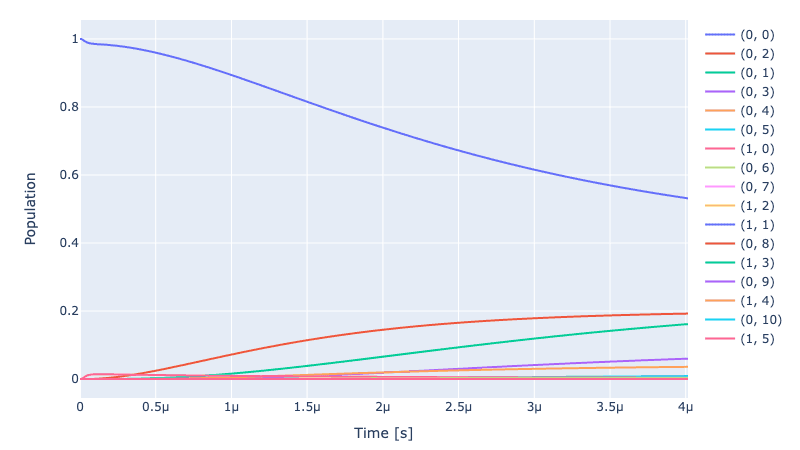$\textit{\textrm{Fig.2 Population dynamics of the mixed (buffer,cat) modes during unoptimized state-preparation with a 4}} \mu s \textit{\textrm{ rectangular drive pulse.}}$

### 2.1.4. Wigner visualisation of unoptimized cat-state preparation

Wigner visualisation of unoptimized cat-state evolution during state preparation:

catprep_pt = get_partial_trace(sequence, initial_state, simulation, buffer, cat) # first goes buffer mode, then cat
wv = OscillatorStateViewer(catprep_pt) # state evolution of cat modes for given sequence
wv.show()


$\textit{\textrm{Video.1 Wigner visualisation of the unoptimized cat-state evolution during state preparation.}}$

To check the expectation value of the cat-mode and the buffer-mode throughout the time $\textcolor{orange}{\textrm{evolution}}$, we evaluate the expectation value of the Hamiltonian operator of the cat cavity and the buffer resonator, and plot them below.

# Calculate the mean value operator through time
cat_number_t = simulation.evolution(sequence, initial_state, operator=cat.get_expectation())
# Plot mean value through time for the cat mode
plot_evolution(sim_res, np.asarray(cat_number_t))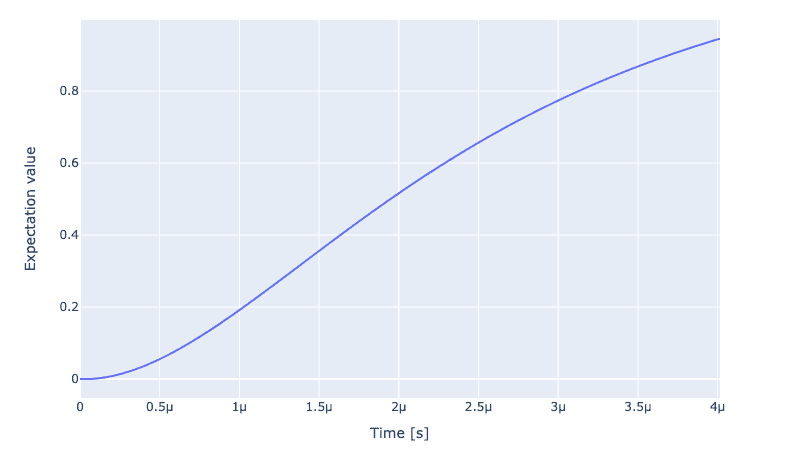$\textit{\textrm{Fig.3 Unoptimized dynamics: Expectation value of the cat-cavity as a function of drive time.}}$

The cavity starts from $0$ photon occupation number and becomes populated to some $|\alpha|^2$ after being pumped. It does not reach the targeted photon number of $|\alpha|^2=2.25$ within the total drive time.

On the other hand, the buffer starts from ground state and then its population peaks quickly due to the drive, and then it dissipates immediately due to its short $t_1$ life span. It saturates to a non-zero occupation number due to the anharmonicity term in its Hamiltonian.

View the unoptimized final cat-state after creation

# Get the final propagator (single value)
final_state = simulation.dynamics(sequence, initial_state)[-1]
final_dm = _partial_trace(final_state, buffer, cat) # partial trace for extracting cat mode
wv = OscillatorStateViewer(final_dm)
wv.show()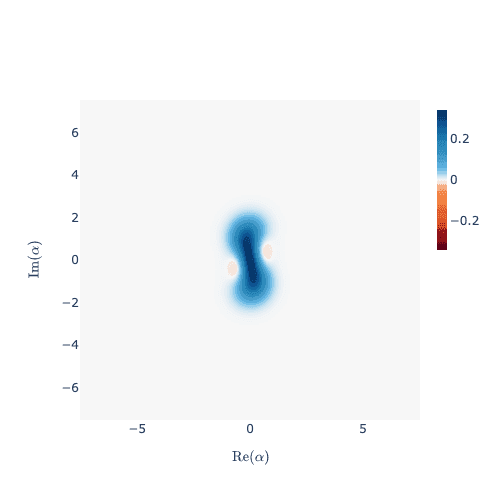$\textit{\textrm{Fig.4 Wigner map of unoptimized final cat-state after state preparation.}}$

We also create an ideal cat-state with even parity that we will use later for the optimization purpose.

View the ideal final cat-state:

cat_phase = 1j if tf.math.sign(driveB_amp) == 1.0 else 1 # this is the phase info for cat-state based on sign of driveB_amp
target_alpha = copy.deepcopy(alpha) # np.sqrt(max(np.asarray(cat_number_t))) # value obtained from the saturation plot of the cat-mode expectation value vs time
ideal_final_cat_state = cat_state_vec(odd_or_even="even", alpha=target_alpha*cat_phase, hilbert_dim=dim_cat)
wv = OscillatorStateViewer(ideal_final_cat_state)
wv.show()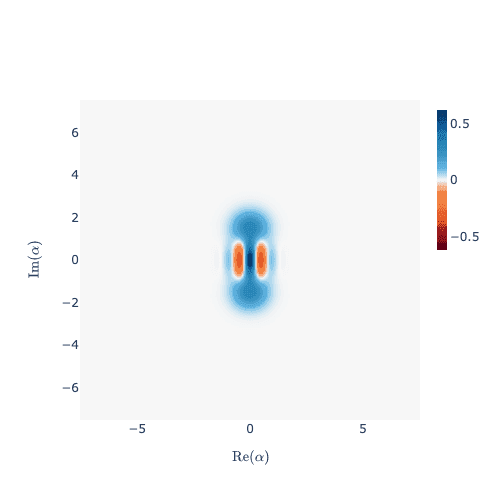$\textit{\textrm{Fig.5 Desirable ideal even cat-state corresponding to the target photon occupation number 2.25 in the cat-cavity.}}$

### 2.1.5. Optimal Control for state preparation

Quantum optimal control is a method for improving the performance of quantum gates, which are the fundamental building blocks of quantum computation. One way to do this is by using algorithms such as the GRAPE (Gradient Ascent Pulse Engineering) algorithm, which uses gradient ascent to find the optimal control pulse for a given quantum gate. This can be used to improve the fidelity of the gate, which is a measure of how accurately it performs its intended operation.

By finding the optimal control pulse, we can reduce errors and improve the overall performance of the quantum computation. The GRAPE algorithm has been successfully applied to various quantum systems, including trapped ion quantum computing, and can be a powerful tool for improving the performance of quantum gates.

We start by introducing a parameter map for the optimization, defining the parameters that can be tuned. In this case, we will adjust the drive amplitude and pulse shapes for the buffer and the cat-cavity.

Open-loop optimal control for generator pulses

Now, open-loop optimisation is set up with a new set of $\textcolor{orange}{\textrm{Instructions}}$.

opt_model = copy.deepcopy(model)

drive_opt = gates.Instruction(
name="OptimalDrive",
channels=["dB", "dC"],
t_start=0.0,
t_end=t_driveB
)


Define a piece-wise-linear shape for the drive pulses

n_bins = 300 # number of bins for dividing pwl pulse shape, try increasing

# Sample params for optimizing drive for buffer
sampled_dB_params = {
"t_sampled": Qty(value = np.linspace(0, t_driveB, n_bins)),
"inphase": Qty(
value = driveB_amp * np.ones(n_bins),
min_val = 0,
max_val = 8*driveB_amp,
unit="Hz 2pi"
),
"t_final": Qty(value = t_driveB, unit="s")
}
sampled_dB_pulse = pulse.Envelope(name="opt-drive-buffer", params=sampled_dB_params, shape=envelopes.pwl_shape_sampled_times)

# Sample params for optimizing drive for cat
sampled_dC_params = {
"t_sampled": Qty(value = np.linspace(0, t_driveB, n_bins)),
"inphase": Qty(
value = driveC_kerr_correct * np.ones(n_bins),
min_val = 8*driveC_kerr_correct,
max_val = 0,
unit="Hz 2pi"
),
"t_final": Qty(value = t_driveB, unit="s")
}
sampled_dC_pulse = pulse.Envelope(name="opt-drive-cat", params=sampled_dC_params, shape=envelopes.pwl_shape_sampled_times)

# Add drive components to the Instructions


Set up the parameter map

opt_map = PMap(instructions=[drive_opt], model=model, generator=genr)
# Declare which parameters needs optimizing
drive_opt_map = [
[(drive_opt.get_key(), "dB", "opt-drive-buffer", "inphase")],
[(drive_opt.get_key(), "dC", "opt-drive-cat", "inphase")],
]

opt_map.set_opt_map(drive_opt_map)
opt_exp = Exp(pmap = opt_map, sim_res=sim_res, prop_method="Tsit5") # We have dissipation turned on, hence Tsit5 prop_method


System Dynamics (Pre-Optimization)

sequence = [drive_opt.get_key()]
opt_exp.set_opt_gates_seq([sequence])
result = opt_exp.compute_propagators()


Pulse Optimisation for cat-state preparation

We then optimize the drive amplitude. As a fidelity function, we choose the density matrix overlap measurement as $F_{cat} = Re(Tr[ \sqrt{ρ_{ideal}} \cdot ρ_{simulated} \cdot \sqrt{ρ_{ideal}} ])$ corresponding to the cat-states and the buffer states, as the ideal target buffer state should be a 3-level ground state. So the final goal function is $1- F_{cat} \cdot F_{buffer}$. We also choose L-BFGS (a gradient-based optimisation algorithm that we wrap from scipy) from our library.

log_dir = os.path.join(tempfile.TemporaryDirectory().name, "qruise-logs")

# Further arguments for the fidelity calculation
fid_func_kwargs = {
"initial_dm": tf_utils.tf_state_to_dm(initial_state), # since we are using Lindbladian evolution, hence dm
"ideal_dm": tf_utils.tf_state_to_dm(ideal_final_cat_state), # ideal_final even cat state dm,
"cavity1": buffer, # need to mention component class for partially tracing out cat-modes
"cavity2": cat, # need to mention component class for partially tracing out cat-modes
}

# Set up OptimalControl
opt = OptimalControl(
dir_path=log_dir,
fid_func=dm_overlap_infid,
fid_subspace=["buffer", "cat"],
pmap=opt_map,
algorithm=algorithms.lbfgs,
options={"maxfun": 25, "ftol": 1e-8, "gtol": 1e-8},
run_name="better-drive",
logger=[PlotLineChart(ylim=[0, 1])],
fid_func_kwargs=fid_func_kwargs,
)


Set experiment, run optimizer, and view results

opt.set_exp(opt_exp)
opt.optimize_controls()
print(opt.current_best_goal)

Output: 0.4282182819282024


The optimized pulse shapes:

genr.draw(drive_opt, resolution=sim_res, centered=False)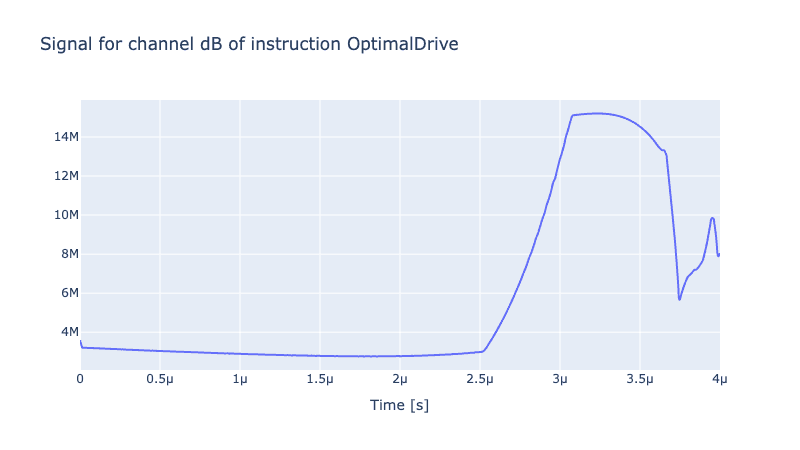$\textit{\textrm{Fig.6 Optimized generator pulses driving the buffer for cat-state preparation in the cat-cavity.}}$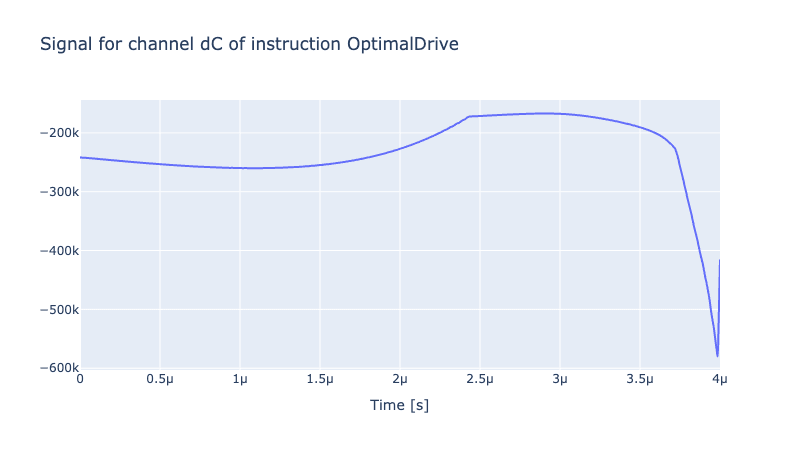$\textit{\textrm{Fig.7 Optimized generator pulses for Kerr-control on the cat-cavity during cat-state preparation.}}$

Results of the optimisation Plotting the dynamics with the initial ground state:

sequence = [drive_opt.get_key()]
result = opt_exp.compute_propagators()
opt_exp.draw_dynamics(sequence, initial_state)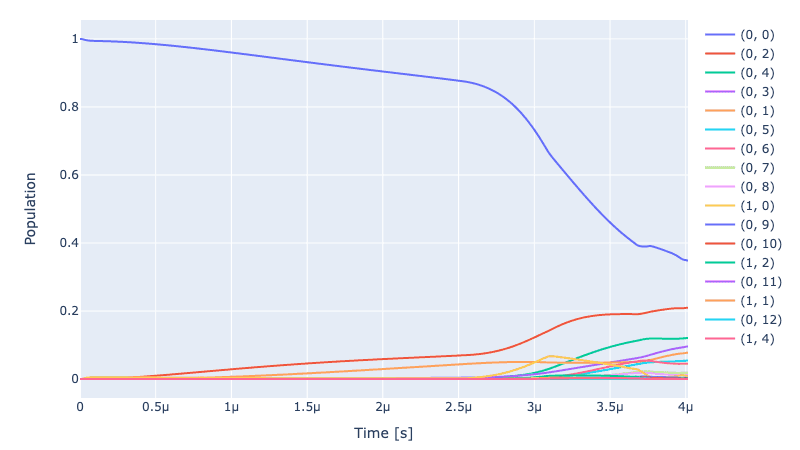$\textit{\textrm{Fig.8 Optimized population dynamics of mixed (buffer,cat) modes during cat-state preparation.}}$

View the optimized final cat-state after cat-creation:

# Get the final propagator (single value)
optimized_final_dm = opt_exp.dynamics(sequence, initial_state)[-1]

optimized_final_dm = _partial_trace(optimized_final_dm, buffer, cat) # partial trace for extracting cat mode
wv = OscillatorStateViewer(optimized_final_dm)
wv.show()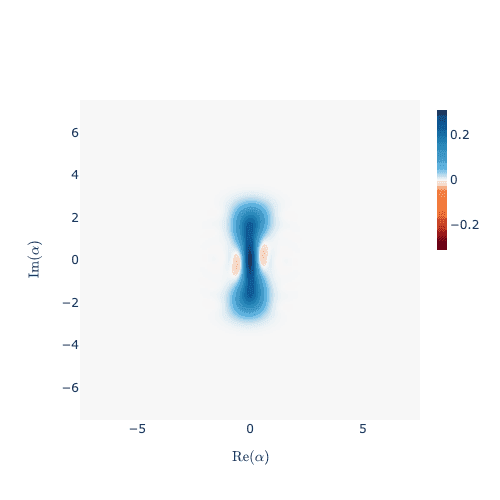$\textit{\textrm{Fig.9 Optimized final cat-state after state preparation.}}$

# Calculate and view the expectation value operator through time
opt_cat_number_t = opt_exp.evolution(sequence, initial_state=initial_state, operator=cat.get_expectation())
plot_evolution(sim_res, np.asarray(opt_cat_number_t))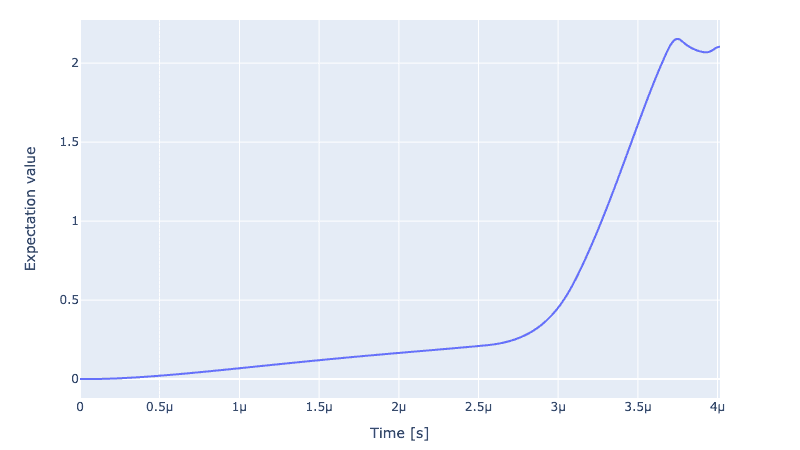$\textit{\textrm{Fig.10 Optimized expectation value of the cat-cavity as a function of time.}}$

The cavity starts from $0$ photon occupation number and becomes populated to $|\alpha|^2=2.16$ after being pumped, which is $96\%$ of the targeted photon number $2.25$. This is a substantial improvement compared to the state-preparation with unoptimized rectangular drive pulses acting on the buffer and cat modes, leading to only $|\alpha|^2=0.8$ during the same drive duration.

### 2.1.6 State preparation fidelity

The state preparation fidelity is defined as the overlap between the prepared state and the ideal cat state, $F_{cat} = Re(Tr[ \sqrt{ρ_{ideal}} \cdot ρ_{simulated} \cdot \sqrt{ρ_{ideal}} ])$. The state evolution Hamiltonian is parity preserving, hence for an initial vacuum state with even parity, the final ground state of the Hamiltonian is an even cat-state $|C^+_\alpha \rangle$. For an initial odd parity excited state occupying the first Fock level, the final ground state of the Hamiltonian is an odd cat-state $|C^-_\alpha \rangle$. As our initial state was set to vacuum, the ideal (target) cat-state density matrix is defined as $ρ_{ideal} = |C^+_\alpha \rangle \langle C^+_\alpha |$ where $|C^+_\alpha \rangle = \frac{1}{\sqrt{2}} (| \alpha \rangle + | - \alpha \rangle)$, $\alpha$ being the coherent state amplitude.

Although optimal control of drive pulses is supposed to enhance fidelity of state preparation and its efficiency in terms of pulse duration etc., hardware limitations can be a bottleneck of the process. The cat-buffer coupling strength $g_2$ and the single-photon loss rate $\kappa_1$ are two such important parameters of the hardware that affects the state preparation fidelity. Hence, we explored state preparation fidelity measure as a function of $g_2$ and $\kappa_1$, before and after Qruise Optimal Control (QOC) software was used. The results are shown in Fig.11 below.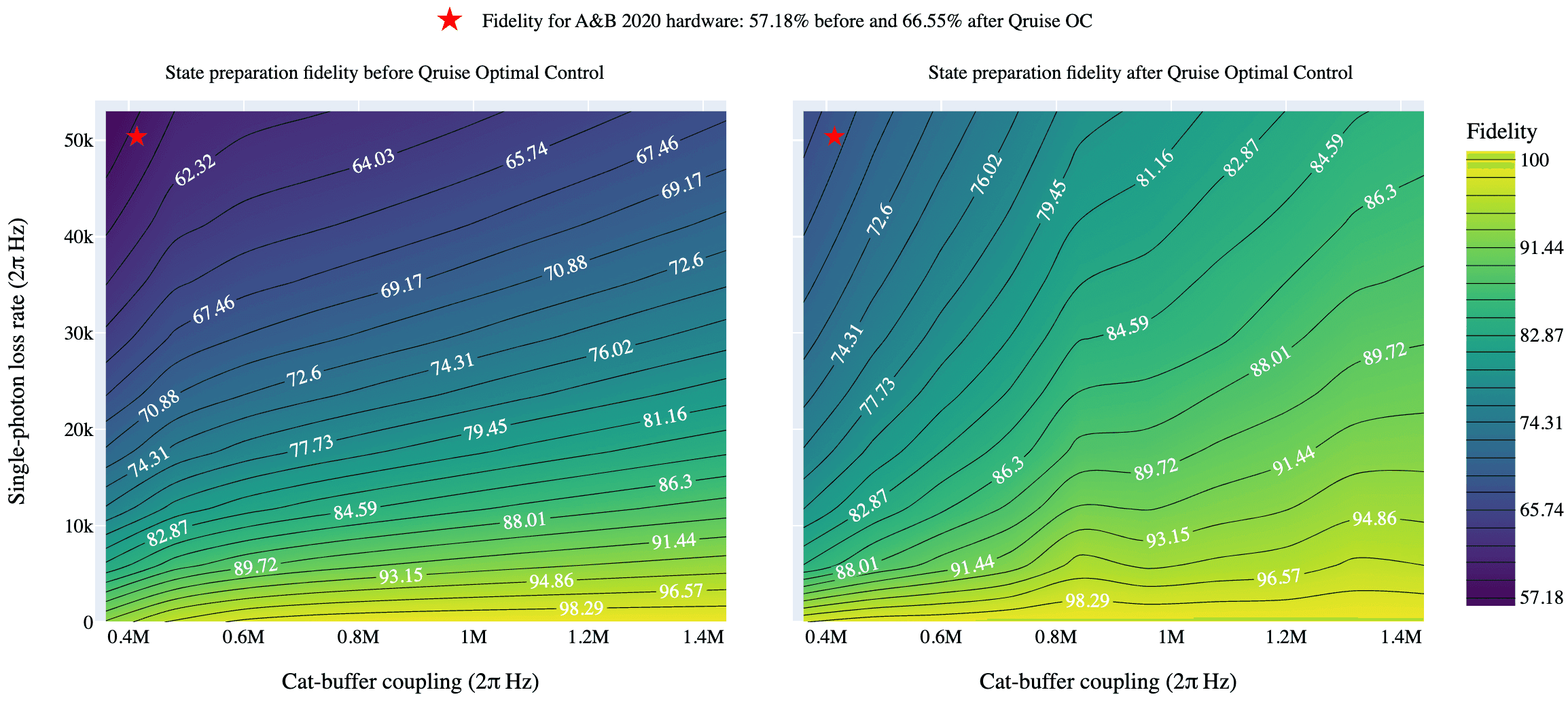$\textit{\textrm{Fig.11 State preparation fidelity as a function of cat-buffer coupling value and single-photon loss rate, before and after Optimal control.}}$

The subplot on the left (right) shows a contour map of the state preparation fidelity before (after) QOC. Ideally, very large cat-buffer coupling strength $g_2$ and zero single-photon loss rate $\kappa_1$ is desired for high fidelity state preparation. However, a relatively low $g_2=0.36$ MHz and non-zero $\kappa_1=53$ kHz of the hardware (marked with a red star in both the subplots) severely limits the unoptimized state preparation fidelity to $57.18$%. After QOC, the fidelity is improved to $66.55$%. As one increases $g_2$ and decreases $\kappa_1$, the QOC protocol drastically improves the state preparation fidelity, often improving by a factor of $1.2$ or more, surpassing $98$% for certain values of $g_2$ and $\kappa_1$. This is qualitatively evident in the contour plot on the left showing darker blue regions of lower fidelity values, while the contour plot on the right shows lighter green regions of higher fidelity values, where the colorscale is indicated by the legend in the right. We show that the QOC software is partially able to compensate for the loss of fidelity due to the hardware limitations.

Future experimental efforts directed at achieving higher $g_2$ and lower $\kappa_1$, via better hardware design, can further improve the state preparation fidelity with the help of Qruise Optimal Control software.

In the next section, we will continue discussing the Wigner tomography protocol for extracting the cat-state from a cat-transmon system, for the same hardware parameters as used above for state preparation.

## 2.2. Wigner tomography protocol

The following section simulates the Wigner tomography protocol for extracting a cat-state, by reading out a transmon coupled to the cat cavity via a cross-Kerr term $\chi_{qa}$. The corresponding Hamiltonian in the frame of the cat-transmon mode is:

\begin{aligned} \mathbf{H}_{RWA} /\hbar = &\omega_{a,0} \mathbf{a^† a} + \omega_{q,0} \mathbf{q^† q} - \sum_{m=a,q} \frac{\chi_{mm}}{2}\mathbf{m}^{†2}\mathbf{m}^{2} - \chi_{qa}\mathbf{a}^{†}\mathbf{a}\mathbf{q}^{†}\mathbf{q} + \epsilon_q(t) (\mathbf{q^†} + \mathbf{q}) + \epsilon_C(t) \mathbf{a^†} \mathbf{a} \end{aligned}

where $\mathbf{a/q}$ are annihilation operators of cat/transmon modes, $\omega_{a/q,0}$ their resonant frequencies. $\chi_{aa/qq}$ is the Kerr term (anharmonicity) for the cat/transmon resonator, and $\chi_{qa}$ is the cross-Kerr coupling between them. $\epsilon_q(t)$ is the drive function coupled to the transmon-mode corresponding to gate applications. $\epsilon_C(t)$ is the drive function coupled to the cat-mode to enable Kerr-effect correction.

The protocol:

After a cat/coherent-state has been created in the cat-cavity, one applies an $R_y(\pi/2)$ gate to the transmon, waits for $\pi/\chi_{qa}$ secs, followed by another $R_y(\pi/2)$ gate on the transmon. During the delay time, the mixed cat-transmon mode evolve under the cross-Kerr coupling that acts like a controlled phase rotation, with the cat-qubit as the target. At the end of this protocol, one reads out the transmon mode by post-selecting on its ground (or excited) state. The corresponding coefficient of this ground (or excited) state gives a measure of the odd (or even) cat state. This protocol is essentially a Ramsey-type measurement of the transmon, with an additional coupling to the cat-cavity.

The transmon gate time durations are ~$ns$, whereas the delay time due to $\pi/\chi_{qa}$ is ~$\mu s$. Hence, we treat the transmon gates as ideal gates applied instantenously, while we use unitary propagation methods for the evolution of the mixed modes during delay/wait times. For the time scales involved in this protocol, the evolution can be essentially treated as dissipation-less, as the cat $t_1$ lifetime is much larger than the relevant gate application times.

Defining ideal gates acting on a 3-level qubit coupled to a cat-cavity:

ry90p_ideal_gate, ry90m_ideal_gate = ideal_3level_Ry_gates(dim_cat)


### 2.2.1. Instantiating transmon mode

We have already defined a "cat" component class before with the Hamiltonian terms $(\omega_{a,0} \mathbf{a^† a} - \frac{\chi_{aa}}{2}\mathbf{a}^{†2}\mathbf{a}^{2})$. We will reuse this in our new model below. Next, we add the transmon-Hamiltonian terms $(\omega_{q,0} \mathbf{q^† q} - \frac{\chi_{qq}}{2}\mathbf{q}^{†2}\mathbf{q}^{2})$ using the component class Qubit:

# Transmon
transmon = components.Qubit(
name="transmon",
desc="Transmon mode",
freq=Qty(value=freq_trans, unit="Hz 2pi"),
anhar=Qty(value=chi_trans, unit="Hz 2pi"),
hilbert_dim=dim_trans,
t1=Qty(value=t1_trans, unit="s"),
t2star=Qty(value=t2_trans, unit="s"),
temp=Qty(value=qubit_temp, unit="K"),
)


We add the cross-Kerr coupling between the cat and transmon-mode $(\chi_{qa}\mathbf{a}^{†}\mathbf{a}\mathbf{q}^{†}\mathbf{q})$.

# Cross-kerr coupling between transmon and cat cavity
kerr_cat_trans = components.Coupling(
name="cat-transmon kerr",
desc="cross-kerr cat and transmon",
connected=["transmon", "cat"],
strength=Qty(value=chi_trans_cat, unit="Hz 2pi"),
hamiltonian_func=hamiltonians["cross_kerr"],
)


### 2.2.2. Setting up drive Instructions

Next, for gate applications on the transmons, we set up the drive function $\epsilon_q(t)$ with its associated Hamiltonian term $(\mathbf{q^†} + \mathbf{q})$.

drive_trans = components.Drive(
name="dT",
desc="Drive transmon",
comment="Drive line 2 on transmon",
connected=["transmon"],
hamiltonian_func=hamiltonians["x_drive"],
)


Next, we set up a drive function $\epsilon_C(t)$ with its associated Hamiltonian term $(\mathbf{a^†} \mathbf{a})$ acting on the cat-cavity as a corrective term for Kerr-effect.

# Driving the cat for Kerr-correction
WT_drive_cat = components.Drive(
name="WT_dC",
desc="Drive cat",
comment="Drive line 2 on cat",
connected=["cat"],
hamiltonian_func=hamiltonians["cat_mode"],
)


### 2.2.3. Mapping Parameters and instantiating tomography Experiment

Next, we create the Model, the Generator, the Instructions, the parameter map, and the Experiment.

Creating Model:

# Model
WT_model = Mdl(
subsystems=[transmon, copy.deepcopy(cat)],  # Individual, self-contained components, first goes transmon mode, then cat
couplings=[kerr_cat_trans, drive_trans, WT_drive_cat],  # Interactions between components
von_neumann=True, # using von_neumann since our input state will be a density matrix
dressed=False,
use_FR=False
)


Creating Generator for gate application, chained with the drive on the transmon "dT" and the cat "WT_dC":

WT_generator = Gnr(
devices={
"LO": devices.LO(name="lo"),
"AWG": devices.AWG(name="awg", resolution=awg_res),
"DigitalToAnalog": devices.DigitalToAnalog(name="dac"),
"Mixer": devices.Mixer(name="mixer"),
"VoltsToHertz": devices.VoltsToHertz(
name="v_to_hz",
V_to_Hz=Qty(value=v2Hz, min_val=0.9*v2Hz, max_val=1.1*v2Hz, unit="Hz/V"),
),
},
chains={
"dT": {
"LO": [],
"AWG": [],
"DigitalToAnalog": ["AWG"],
"Mixer": ["LO", "DigitalToAnalog"],
"VoltsToHertz": ["Mixer"],
},
"WT_dC": {
"LO": [],
"AWG": [],
"DigitalToAnalog": ["AWG"],
"Mixer": ["LO", "DigitalToAnalog"],
"VoltsToHertz": ["Mixer"],
},
},
)


For the delay time $t_{delay}$, we create a pulse without any driving envelope.

Organizing Drive pulses with no_drive envelope:

nodrive_env = pulse.Envelope(
name="no_drive",
params={"t_final": Qty(
value=t_delay,
min_val=0.5 * t_delay,
max_val=1.5 * t_delay,
unit="s"
)
},
shape=envelopes.no_drive,
)


For the drive on the cat-cavity for Kerr-effect correction, the amplitude of the drive function on the cat-cavity should be set to the targeted $|\epsilon_C| = \chi_{aa} (2 |\alpha| ^2 +1)$ to enable correction of Kerr-effect.

Organizing Drive pulses with square envelope for cats:

# Amplitude of the drive for cat
square_params = {
"amp": Qty(value=driveC_kerr_correct, unit="Hz 2pi"),
"t_final": Qty(value=t_delay, unit="s")
}
square_amp_cat = pulse.Envelope(
name="square",
shape=envelopes.rect ,
params=square_params
) # optimize this

# Carrier frequency of the drive for cat cavity
square_freq = pulse.Carrier(
name="carrier",
desc="Frequency of the local oscillator",
params={"freq": Qty(value=0, unit="Hz 2pi")},
)


Creating carrier frequency for the Instruction:

# drive set up for transmon gates
lo_freq_q1 = freq_trans + sideband
carrier_parameters = {
"freq": Qty(
value=lo_freq_q1,
unit="Hz 2pi"
),
"framechange": Qty(
value=0.0, min_val=-np.pi, max_val=3 * np.pi, unit="rad"
),
}
carr = pulse.Carrier(
name="carrier",
desc="Frequency of the local oscillator",
params=carrier_parameters
)


Creating Instructions for delay/wait time as an identity gate on the transmon:

QId = gates.Instruction(
name="transmon Id",
t_start=0.0,
t_end=t_delay,
channels=["dT", "WT_dC"],
)

# adding the transmon drive for gates in the instructions
QId.comps["dT"]["carrier"].params["framechange"].set_value(
(-sideband * t_delay) % (2 * np.pi)
)

# adding the cat drive in the instructions


Create Parameter map:

# Parameter map
WT_parameter_map = PMap(
instructions=[QId],
model=WT_model,
generator=WT_generator
)


Create Experiment:

# Experiment
WT_simulation = Exp(pmap=WT_parameter_map, sim_res = sim_res, prop_method="Tsit5")


Combining instantenous gates with instructions:

We create a function to compute the propagator for our Instruction, followed by the method "combine", where we apply an ideal gate before and after the evolution of the instruction. We update the 'exp.propagator' with this modified instruction.

def combine_instantenous_gates(experiment, instruction, ideal_gate, name):

opt_id_propagator = experiment.compute_propagators()[instruction.get_key()]
opt_id_propagator = opt_id_propagator.combine(
ideal_gate,
name=name,
convert_to_super=True,
apply_at_end = True,
apply_at_start=True
)
experiment.propagators[instruction.get_key()] = opt_id_propagator

return experiment


Using the function above, we compute the propagator for our Instruction (which is just an identity gate), followed by the method "combine", where we apply the "ry90p" gate before and after the evolution of the instruction. We update the propagator with this modified instruction.

WT_simulation = combine_instantenous_gates(WT_simulation, QId, ry90p_ideal_gate, name="ry90p")


We create an initial_state out of Kronecker product between the 3-level transmon ground state and the prepared cat-state from earlier state-prep. Note that the order of the Kronecker product here follows the order of the subsystems introduced in the Model constructor above, which in this case is the transmon mode followed by the cat-mode.

Create the initial state for Wigner Tomography sequence

dm_cat = optimized_final_dm # this is output cat dm from state-prep earlier
psi_trans = np.asarray([ * dim_trans]).T
psi_trans = 1 # ground state of transmon
dm_trans = _return_dm(psi_trans) # converting vector to a density matrix

cat_transmon_dm = np.kron(dm_trans, dm_cat) # first goes transmon mode, then cat
cat_transmon_dm = tf.cast(cat_transmon_dm, dtype=tf.complex128)


Next, we create a specific sequence following our Instructions, and evolve a specific initial state based on this sequence.

# evolve the initial state based on the oscillations
sequence=[QId.get_key()]


One can take partial trace out of the mixed cat-transmon modes to extract the cat states. Then one can plot the Wigner visualization of the cat-state evolution.

### 2.2.4. Wigner visualization of unoptimized cat-state evolution during tomography

We can visualize the Wigner map of the unoptimized cat-state evolution during Wigner tomography:

cat_pt = get_partial_trace(sequence, cat_transmon_dm, WT_simulation, transmon, cat) # first goes transmon mode, then cat
wv = OscillatorStateViewer(cat_pt) # state evolution of cat modes for given sequence
wv.show()


$\textit{\textrm{Video. 2 Cat-state evolution during unoptimized Wigner tomography.}}$

Due to Kerr-effect, we obtain a cat-state that gets distorted during the tomography protocol.

Post-select on ground or excited state of transmon:

Next, we post-select on the ground (g) or excited (e) state of the transmon in the final state after the evolution, to read out the odd or even cat-state of the cat-qubit.

final_state = WT_simulation.dynamics(sequence, cat_transmon_dm)[-1]
post_odd = post_select_state(g_or_e="g", transmon_mode=transmon, cat_mode=cat, final_state=final_state)
post_even = post_select_state(g_or_e="e", transmon_mode=transmon, cat_mode=cat, final_state=final_state)


View the unoptimized odd-cat state after tomography:

wv = OscillatorStateViewer(post_odd) # final odd cat state from post-selection on ground state of the transmon qubit
wv.show()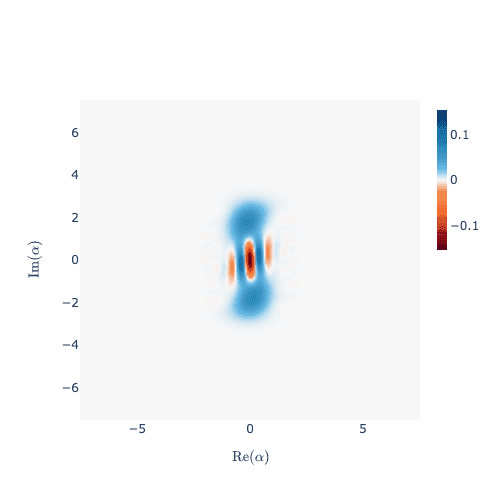$\textit{\textrm{Fig.12 Unoptimized odd-cat state after tomography.}}$

View the unoptimized even-cat state after tomography:

wv = OscillatorStateViewer(post_even) # final even cat state from post-selection on excited state of the transmon qubit
wv.show()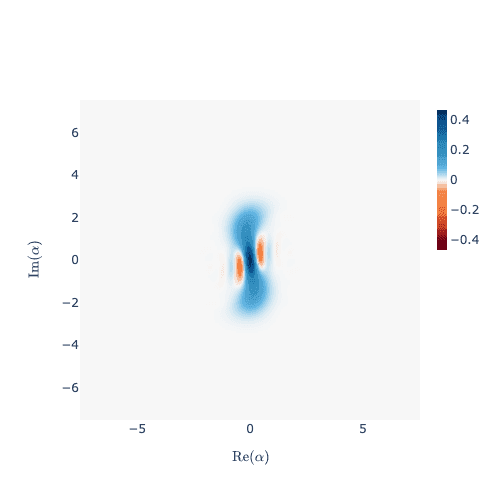$\textit{\textrm{Fig.13 Unoptimized even-cat state after tomography.}}$

Due to Kerr-effect, we find the final even cat-state a bit distorted than what the ideal state should have been.

### 2.2.5. Optimal control for generator pulses used in tomography

As was done during state preparation, one can optimize the pulse shape applied on the cat-cavity to account for Kerr-effect correction to the cat-qubit. For this purpose, open-loop optimisation is set up with a new set of instructions.

opt_WT_model = copy.deepcopy(WT_model)

WT_drive_opt = gates.Instruction(
name="OptimalDrive for cats",
channels=["dT", "WT_dC"],
t_start=0.0,
t_end=t_delay
)


Define a piece-wise-linear shape for the drive pulses:

n_bins = 50 # number of bins for dividing pwl pulse shape

# Sample params for optimizing drive for cat
sampled_dC_params = {
"t_sampled": Qty(value = np.linspace(0, t_delay, n_bins)),
"inphase": Qty(
value = driveC_kerr_correct * np.ones(n_bins),
min_val = 5*driveC_kerr_correct,
max_val = 0,
unit="Hz 2pi"
),
"t_final": Qty(value = t_delay, unit="s")
}
sampled_dC_pulse = pulse.Envelope(name="opt-drive-cat", params=sampled_dC_params, shape=envelopes.pwl_shape_sampled_times)


Add drive components to the Instructions:

# adding the transmon drive for gates in the instructions
WT_drive_opt.comps["dT"]["carrier"].params["framechange"].set_value(
(-sideband * t_delay) % (2 * np.pi)
)

# adding the cat drive in the instructions


Set up the parameter map

opt_WT_map = PMap(instructions=[WT_drive_opt], model=opt_WT_model, generator=copy.deepcopy(WT_generator))


Declare which parameters needs optimizing

drive_opt_WT_map = [
[(WT_drive_opt.get_key(), "WT_dC", "opt-drive-cat", "inphase")]
]

opt_WT_map.set_opt_map(drive_opt_WT_map)
opt_WT_exp = Exp(pmap = opt_WT_map, sim_res=sim_res, prop_method="Tsit5")


System Dynamics (Pre-Optimization)

Note that exp.compute_propagators() below will overwrite any extra instructions (such as instantenous gates) from the propagators' unitaries. Hence, we can safely ignore the usage of "combine_instantenous_gate" function for the optimization purpose, as these instantenous gates don't affect the cat-dynamics anyway.

sequence = [WT_drive_opt.get_key()]
opt_WT_exp.set_opt_gates_seq([sequence])
result = opt_WT_exp.compute_propagators() # this overwrites any initial and final instantenous gates


#### Pulse Optimisation for Kerr correction:

We then optimize the drive amplitude. As a fidelity function, we choose the state overlap infidelity measurement as $F = 1-\textrm{Re} (\textrm{Tr} [ ρ_{simulated} . ρ_{ideal} ])$ corresponding to the cat-states. We also choose L-BFGS (a gradient-based optimisation algorithm that we wrap from scipy) from our library.

log_dir = os.path.join(tempfile.TemporaryDirectory().name, "qruise-logs")

# Further arguments for the fidelity calculation
fid_func_kwargs = {
"initial_dm": cat_transmon_dm, # since we are using Lindbladian evolution, hence dm
"ideal_dm": tf_utils.tf_state_to_dm(ideal_final_cat_state), # ideal_final is a kronecker between buffer ground and even cat state vector,
"cavity1": transmon, # need to mention component class for partially tracing out cat-modes
"cavity2": cat, # need to mention component class for partially tracing out cat-modes
}

# Set up OptimalControl
WT_OC = OptimalControl(
dir_path=log_dir,
fid_func=state_overlap_infid, # F = 1- Re (Tr [ ρ_{simulated} . ρ_{ideal} ]) for cat-states
fid_subspace=["transmon", "cat"],
pmap=opt_WT_map,
algorithm=algorithms.lbfgs,
options={
"maxfun": 50,
"ftol": 1e-8,
"gtol": 1e-8
},
run_name="better-drive",
logger=[PlotLineChart(ylim=[0, 1])],
fid_func_kwargs=fid_func_kwargs,
)


Set experiment, run optimizer, and view results

WT_OC.set_exp(opt_WT_exp)
WT_OC.optimize_controls()
print(WT_OC.current_best_goal)

Output: 0.4094454314048629


The optimized pulse shape for Kerr-effect correction on the cat-cavity:

WT_generator.draw(WT_drive_opt, resolution=sim_res, centered=False)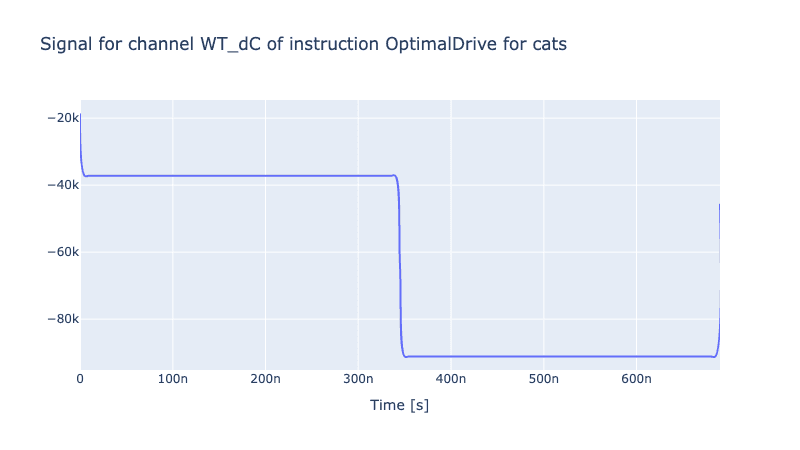$\textit{\textrm{Fig.14 Optimized generator pulses on the cat-cavity for Kerr-control during Wigner tomography protocol.}}$

Results of the optimisation:

sequence = [WT_drive_opt.get_key()]
result = opt_WT_exp.compute_propagators()
opt_WT_exp = combine_instantenous_gates(opt_WT_exp, WT_drive_opt, ry90p_ideal_gate, name="opt_ry90p") # we add this combination again


View the optimized final cat-state:

Next, we post-select on the ground (g) or excited (e) state of the transmon in the final state after the evolution, to read out the odd or even cat-state of the cat-qubit.

opt_final_state = opt_WT_exp.dynamics(sequence, cat_transmon_dm)[-1]
opt_odd = post_select_state(g_or_e="g", transmon_mode=transmon, cat_mode=cat, final_state=opt_final_state)
opt_even = post_select_state(g_or_e="e", transmon_mode=transmon, cat_mode=cat, final_state=opt_final_state)


View the optimized odd-cat state after tomography:

wv = OscillatorStateViewer(opt_odd) # final odd cat state from post-selection on ground state of the transmon qubit
wv.show()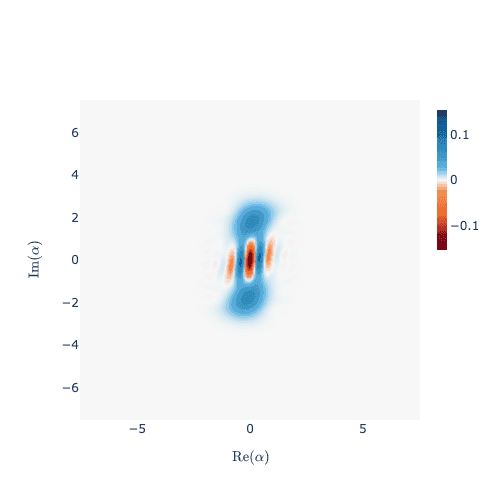$\textit{\textrm{Fig.15 Optimized odd-cat state after tomography.}}$

View the optimized even-cat state after tomography:

wv = OscillatorStateViewer(opt_even) # final even cat state from post-selection on excited state of the transmon qubit
wv.show()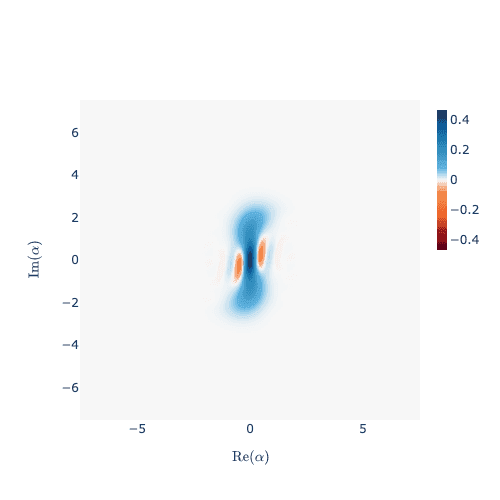$\textit{\textrm{Fig.16 Optimized even-cat state after tomography.}}$

To conclude, we demonstrated optimally controlled state-preparation and Wigner tomography of cat-qubits within the Qruise ecosystem. We prepared a cat-qubit that reaches $96\%$ of a targeted photon occupation number of $2.25$, within $4 \,\mu s$ of pumping and has been Kerr-corrected throughout the state evolution. We also explored state preparation fidelity before and after optimal control, as a function of two important hardware parameters: cat-buffer coupling strength $g_2$ and single-photon loss rate $\kappa_1$. Then, we proposed high-fidelity state-preparation protocol by achieving higher $g_2$ and lower $\kappa_1$ in future experiments, in combination with Qruise Optimal Control software.

Stay tuned for better gate fidelities of bosonic-state models within Qruise toolset! Coming soon ...

## 3. References

. Raphaël Lescanne, Marius Villiers, Theau Peronnin, Alain Sarlette, Matthieu Delbecq, Benjamin Huard, Takis Kontos, Mazyar Mirrahimi, Zaki Leghtas, "Exponential suppression of bit-flips in a qubit encoded in an oscillator", Nature Physics (2020).

. L. Sun, A. Petrenko, Z. Leghtas, B. Vlastakis, G. Kirchmair, K. M. Sliwa, A. Narla, M. Hatridge, S. Shankar, J. Blumoff, L. Frunzio, M. Mirrahimi, M. H. Devoret, R. J. Schölkopf, "Tracking photon jumps with repeated quantum non-demolition parity measurements", Nature (2014).

Back to all posts